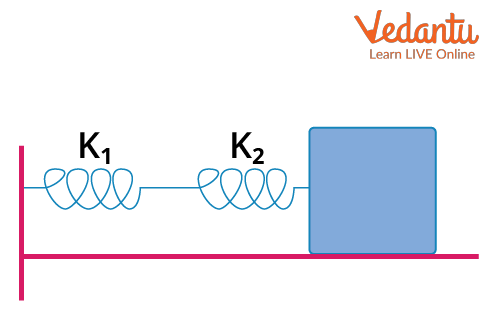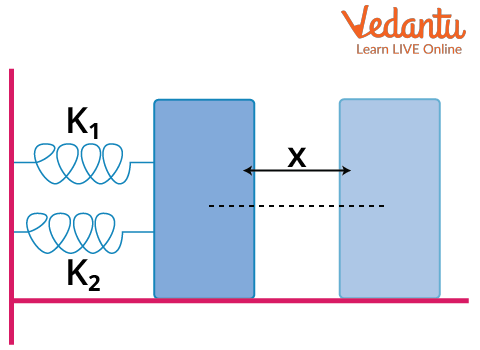Courses
Courses for Kids
Free study material
Free LIVE classes
More

# Series and Parallel Combination of Springs for JEE## Introduction to Simple Harmonic Motion

Last updated date: 20th Mar 2023
Total views: 72.6k
Views today: 1.51k

Periodic motion is the motion that is repeated after a regular interval of time. The period motion is also known as harmonic motion. The period after which the motion is repealed is the time period of the motion. Also, if a body repeats its motion, that is if the body moves to and fro on the same path, then the body is said to be oscillating. These are some basic definitions that you might be familiar with.

So, what is a simple harmonic motion?

Simple harmonic motion is a particular type of oscillation in which the particle accelerates on a straight path. The speciality of this motion is that the acceleration of the particle is always directed towards a fixed point and the magnitude of the acceleration is proportional to the displacement of the particle from this fixed point. The fixed point is known as the centre of oscillation.

## Mathematical Description of SHM

We have already mentioned that the acceleration in an SHM is proportional to the displacement of the particle from the centre of oscillation and is directed toward the centre of oscillation. Suppose the centre of oscillation is at the origin and the particle is executing SHM towards the positive x-axis, then the equation for the simple harmonic motion can be written as:

$a=-\omega^{2} x$

Here, $\omega^{2}$ is a positive constant.

We also know from Newton’s second law that

$a=\dfrac{F}{m}$

We can then write

\begin{align} &\dfrac{F}{m}=-\omega^{2} x \\ &F=-m \omega^{2} x \\ &F=-k x \end{align}

This equation is known as the equation of an SHM and the constant k is called the spring constant. It is to be noted that the force is always opposite to the direction of the motion. This type of force is called restoring force.

A block attached to a spring executes a simple harmonic motion and the equation of SHM can be applied to it. This system is known as a spring-mass system. We will use this equation to find the equivalent spring constants for combinations of springs in series and parallel.

The time period of a spring depends on the angular frequency ω and we can write it as,

$T=\frac{2\pi }{\omega}$

From the above equation of force, we can see that

$m\omega ^{2}= k$

$\omega = \sqrt{\frac{k}{m}}$

Hence, the time period of the spring will

$\omega =2\pi \sqrt{\frac{m}{k}}$

## Series Combination of Springs

Suppose we have a block attached to a system of springs in series combination as shown in the diagram below.Two Springs in Series Combination

The spring constants of the springs are k1 and k2, and they are connected in series.

Suppose we apply a force on the combination of springs through the block. The force on both the springs will be the same. However, the displacement of each spring might be different.

If the spring having spring constant k1 is displaced by x1 and similarly the spring having spring constant k2 is displaced by x2, then the total displacement of both springs can be written as

x = x1 + x2

We know that for spring

F = -kx

Using this we can write

\begin{align} &-\dfrac{F}{k}=\left(\dfrac{-F}{k_{1}}\right)+\left(\dfrac{-F}{k_{2}}\right) \\ &-\dfrac{F}{k}=-F\left(\dfrac{1}{k_{1}}+\dfrac{1}{k_{2}}\right) \\ &\dfrac{1}{k}=\dfrac{1}{k_{1}}+\dfrac{1}{k_{2}} \end{align}

Here, k is the equivalent spring constant of the combination.

Now we know that

\begin{align} &\omega^{2} x=k \\ &\omega=\sqrt{\dfrac{k}{x}} \end{align}

We also know that the time period can be written as

$T=\dfrac{2 \pi}{\omega}$

Combining the equations, we can write

$T=2 \pi \sqrt{\dfrac{m}{k}}$ ....(1)

This time period is also known as the spring time.

Inserting the value of k for the series combination, we get

$T=2 \pi \sqrt{m\left(\dfrac{1}{k_{1}}+\dfrac{1}{k_{2}}\right)}$

This is the time period of the combination.

The formula for the equivalent spring constant can be extended to a system of n springs in a series combination. We can write

$\dfrac{1}{k}=\dfrac{1}{k_{1}}+\dfrac{1}{k_{2}}+\ldots+\dfrac{1}{k_{n}}$

## Parallel Combination of Springs

Suppose we now have a combination of springs in parallel as shown in the figure below.Two Springs in a Parallel Combination

The spring constants are k1 and k2. As it is visible in the figure, the springs are connected in parallel to a block. Let’s suppose that we displace the block by a distance x. The displacement of both the blocks, in this case, will be the same, but the force by each spring will be different. However, we can write the sum of the forces of the combination F as:

F =F1 + F2

Using the equation of SHM, we can write

\begin{align} &-k x=-k_{1} x+\left(-k_{2} x\right) \\ &-k x=-x\left(k_{1}+k_{2}\right) \\ &k=k_{1}+k_{2} \end{align}

This is the equivalent spring constant of the springs in a parallel combination.

The time period of this combination can be found by substituting the value of the equivalent spring constant in equation (1). This gives

\begin{align} &T=2 \pi \sqrt{\dfrac{m}{k}} \\ &T=2 \pi \sqrt{\dfrac{m}{k_{1}+k_{2}}} \end{align}

We can also generalise the formula for the equivalent spring constant for a combination of n springs in a parallel combination. The equivalent spring constant will then be

$k_{p}=k_{1}+k_{2}+\ldots+k_{n}$

## Numerical Examples on Series and Parallel Combination of Springs

Example 1: Identical springs of spring constant K are connected in series and parallel combinations. A mass m is suspended from them. Find the ratio of the time periods of their vertical oscillation.

Solution:

For series combination, the equivalent spring constant ks will be

\begin{align} &\dfrac{1}{k_{s}}=\dfrac{1}{k}+\dfrac{1}{k} \\ &\dfrac{1}{k_{s}}=\dfrac{2}{k} \\ &k_{s}=\dfrac{k}{2} \end{align}

The time period of this combination will be

$T_{s}=2 \pi \sqrt{\dfrac{2 m}{k}}$

Similarly, the equivalent spring constant kp for the parallel combination will be

\begin{align} &k_{p}=k+k \\ &k_{p}=2 k \end{align}

The time period of this parallel combination will be

$T_{p}=2 \pi \sqrt{\dfrac{m}{2 k}}$

The ratio of TS and TP  will then be

\begin{align} &\dfrac{T_{s}}{T_{p}}=\dfrac{2 \pi \sqrt{\dfrac{2 m}{k}}}{2 \pi \sqrt{\dfrac{m}{2 k}}} \\ &\dfrac{T_{s}}{T_{p}}=\sqrt{\dfrac{2 m}{k} \times \dfrac{m}{2 k}} \\ &\dfrac{T_{s}}{T_{p}}=\sqrt{4} \\ &\dfrac{T_{s}}{T_{p}}=2 \end{align}

The ratio of the time period of the series and the parallel combination is 2:1.

Example 2: The resultant force acting on a particle that is attached to a spring executing simple harmonic motion is 10 N, when it is 2 m away from the centre of oscillation. Find the spring constant.

Solution: The force by the spring is given as

F=-kx

Since we need only the magnitude of k, we can ignore the negative sign which shows us the direction of the force. The magnitude of the spring constant can be written as

\begin{align} &k=\left|\dfrac{F}{x}\right| \\ &k=\left|\dfrac{10}{2}\right| \\ &k=5 \mathrm{Nm}^{-1} \end{align}

The spring constant of the spring is 5 Nm-1.

## Conclusion

A particular kind of oscillation in which the particle accelerates along a straight route is known as simple harmonic motion. The unique feature of this motion is that the particle's acceleration is always pointed in the direction of a fixed point, and the strength of the acceleration is inversely proportional to the particle's displacement from the fixed point. The fixed point is referred to as the oscillation's centre. A spring and mass system executes SHM. The restoring force by a spring having spring constant k and displacement x is given as

F=-kx

The equivalent spring constant of a system of n springs connected in series is given as

$\dfrac{1}{k_{s}}=\dfrac{1}{k_{1}}+\dfrac{1}{k_{2}}+\ldots+\dfrac{1}{k_{n}}$

Similarly, the equivalent spring constant of a system of n springs connected in parallel is given as

$k_{p}=k_{1}+k_{2}+\ldots+k_{n}$

## FAQs on Series and Parallel Combination of Springs for JEE

1. Does the spring constant change with length?

The name spring constant suggests that the value of the spring constant would be constant, but that is not the case. The spring constant diminishes as the spring lengthens. The relationship between the spring constant and length is inverse. The spring constant rises to double its initial value if the spring is reduced to half of its original length. So, while dealing with the numerical problems of springs, it is important to see if the spring constant is given to be constant or if it is changing with the length.

2. What is the weightage of the combination of springs in JEE Main?

There is no separate chapter on springs, and it is covered under the topic of simple harmonic motion. This topic includes other oscillatory type motions like the motion of a simple pendulum, torsional pendulum, physical pendulum etc. Since simple harmonic motion deals with oscillations, it comes under the broad chapter of oscillations and waves. This chapter has a weightage of about 3.3% and every year at least one question is asked from it. So, this is an important topic to cover for JEE Main